Mechanics

# Speed, period and frequency in the MCU

Velocity , period and frequency are the main parameters used to analyze a body that develops uniform circular motion (MCU). Despite being described as a uniform motion, the MCU is an accelerated motion , since, to describe a circular trajectory, the action of a centripetal force on the mobile is necessary.

## Introduction to the MCU

Uniform circular motion is one in which a mobile follows a circular path of constant radius . In this type of movement, the speed module of the mobile does not change, despite the fact that it is an accelerated movement. Acceleration, in this case known as centripetal acceleration , just changes the direction and direction of velocity, whose magnitude is kept constant.

The study of the MCU involves the relationship between spatial quantities and angular quantities , since the trajectory of a mobile in MCU is calculated as an arc of circumference . Furthermore, in the study of the MCU, the angular quantities are represented in radians. In this case, a complete 360º turn corresponds to an angular change of 2π radians.

## Frequency and period in the MCU

Frequency and period are two quantities related to each other and inversely proportional . The frequency of a body that performs MCU serves to tell us the number of complete turns that the body performs in each unit of time. In SI units, frequency is measured in Hz (s -1 ). Therefore, it is the number of complete revolutions per second.

Period is the physical quantity that measures the time required for a mobile to make a complete revolution around a point. The SI unit of period measurement is the second (s). Period and frequency are quantities related by the following formula: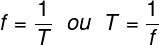T – period (s)

f – frequency (Hz)

## Speed ​​in the MCU

The velocity of a mobile that describes an MCU can be calculated by the ratio between the distance traveled (ΔS) and the time interval of a complete revolution (T). However, it is necessary to remember that the space covered in a complete revolution is the measure of the length of the circle and that the time required to complete it is equal to the period, as shown in the following formula: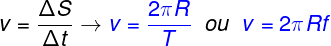v – scalar speed in the MCU (m/s)

In addition to the scalar speed of the MCU, shown above, there is also a speed that we call angular speed. This velocity measures the rate at which angular change occurs and is measured in rad/s . This velocity, denoted by the symbol ω, can be calculated both in terms of period and in terms of frequency.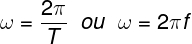## MCU solved exercises

Question 1 — A Ferris wheel of radius 10 m completes a revolution around its center every 30 seconds. Based on this, calculate the speed, in m/s, of the cabins located at the ends of the radius of this Ferris wheel.

a) 2π/3

b) 3π/2

c) π/3

d) 4π/3

Resolution:

To solve the question, it is necessary to find the frequency of the Ferris wheel in Hz. Since it makes one complete revolution every 30 seconds, its frequency is 2 rpm. To convert this frequency into Hz, we divide its modulus by 60. After that, we can apply it to the velocity formula in the MCU.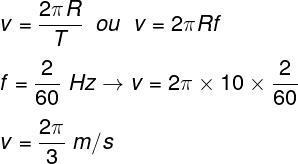Based on the calculation made above, we find that the correct alternative is the letter A .

Question 2 — A chainsaw operates at a frequency of 3600 rpm. Knowing that the diameter of this saw is 20 cm, is the rotation speed of its ends, in km/h, approximately?

Data : Use π = 3.1.

a) 83

b) 245

c) 127

d) 134

Resolution:

As the frequency of the saw is 3600 rpm, we know that it performs 60 complete revolutions per second, so its frequency is 60 Hz. After that, just transform the radius of the saw (10 cm) into meters (0.1 m) and make the calculation below: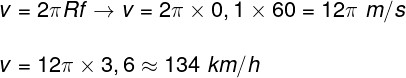Through the calculation made above, we found that the rotation speed of the saw is approximately 134 km/h, so the correct alternative is the letter D.

Question 3 — Planet Earth is located at a distance of approximately 1.5 x 10 8 km from the Sun. Knowing that the Earth’s orbital period, in hours, is approximately 8,770 hours and considering its circular orbit, calculate the translation speed of the Earth around the Sun, in km/h.

Data : use π = 3.1.

a) 30,000 km/h

b) 106,000 km/h

c) 500 km/h

d) 2,500 km/h

Resolution :

To solve the exercise, just use the formula for speed in uniform circular motion.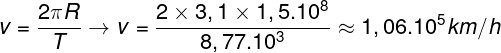According to the calculation, the Earth’s orbital speed is approximately 106,000 km/h. Therefore, the correct alternative is the letter B .